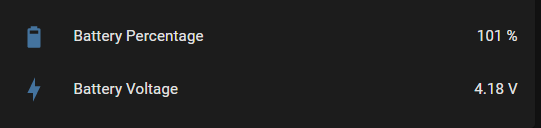# Adafruit MAX17048 LiPo battery gauge with ESPHome

Maybe someone can help, I’m struggling to get this right. I want to use Adafruit’s MAX17048 LiPo battery gauge:

I tried to take inspiration from this thread:

Ok so I tried modifying a-marcel’s code and got it to at least produce some output but the numbers seem wildly wrong:

``````[18:28:14][D][sensor:127]: 'Voltage': Sending state 3616.25000  with 0 decimals of accuracy
[18:28:14][D][sensor:127]: 'Percentage': Sending state 3.10937  with 0 decimals of accuracy
``````

I confess that I really don’t understand what this is doing or why we need all these bit shift operators.

``````  void update() override {
float voltage = 1.25f * (float)(read16(MAX17043_VCELL) >> 4);
voltage_sensor->publish_state(voltage);

float percentage = (float)((percentage_tmp >> 8) + 0.003906f * (percentage_tmp & 0x00ff));

percentage_sensor->publish_state(percentage);
}
``````

Why all the complicated formulas? Does the sensor not produce a simple voltage number and percentage so the data has to be massaged like this?

Ok…so I think I have this solved but maybe someone else can check my work. I copied these formulas from the source code of the Adafruit driver:

So now my code looks like this:

``````  void update() override {
//float voltage = 1.25f * (float)(read16(MAX17048_VCELL) >> 4);
float voltage = (float)(read16(MAX17048_VCELL)) * 78.125 / 1000000;
voltage_sensor->publish_state(voltage);

//float percentage = (float)((percentage_tmp >> 8) + 0.003906f * (percentage_tmp & 0x00ff));
float percentage = (float)(percentage_tmp) / 256;
percentage_sensor->publish_state(percentage);
}
``````

Now it produces outputs that look like a voltage and percentage:

``````[06:24:58][D][sensor:127]: 'Voltage': Sending state 3.56000  with 0 decimals of accuracy
[06:24:58][D][sensor:127]: 'Percentage': Sending state 93.23047  with 0 decimals of accuracy
``````

Does it look right to you? Am I missing anything?

The values are a bit odd, a fully charged battery started at 103% and 4.2V. Methinks my formulas aren’t quite optimized correctly.but at least I can watch the values change as the battery discharges.

This is working great, if anyone else needs a solution for monitoring battery life. Here’s the full code in case anyone else needs it.

MAX17048_component.h - put this file in your esphome folder:

``````#include "esphome.h"

#define MAX17048_VCELL          0x02 // voltage
#define MAX17048_SOC            0x04 // percentage
#define MAX17048_MODE           0x06
#define MAX17048_VERSION        0x08
#define MAX17048_CONFIG         0x0c
#define MAX17048_COMMAND        0xfe

class MAX17048Sensor : public PollingComponent, public Sensor {
public:
Sensor *voltage_sensor = new Sensor();
Sensor *percentage_sensor = new Sensor();

MAX17048Sensor() : PollingComponent(10000) {}

void setup() override {
// Initialize the device here. Usually Wire.begin() will be called in here,
// though that call is unnecessary if you have an 'i2c:' entry in your config
ESP_LOGD("custom", "Starting up MAX17048 sensor");

Wire.begin();
}

uint16_t temp;
Wire.begin();
Wire.write(reg);
Wire.endTransmission();
Wire.endTransmission();
return temp;
}

void update() override {
float voltage = (float)(read16(MAX17048_VCELL)) * 78.125 / 1000000;
voltage_sensor->publish_state(voltage);

float percentage = (float)(percentage_tmp) / 256;
percentage_sensor->publish_state(percentage);
}
};
``````

``````esphome:
includes:
- MAX17048_component.h
``````

and this in your sensor section:

``````  - platform: custom
lambda: |-
auto max17048_sensor = new MAX17048Sensor();
App.register_component(max17048_sensor);
return {max17048_sensor->voltage_sensor, max17048_sensor->percentage_sensor};
sensors:
- name: "Voltage"
unit_of_measurement: V
accuracy_decimals: 2
- name: "Percentage"
unit_of_measurement: '%'
``````